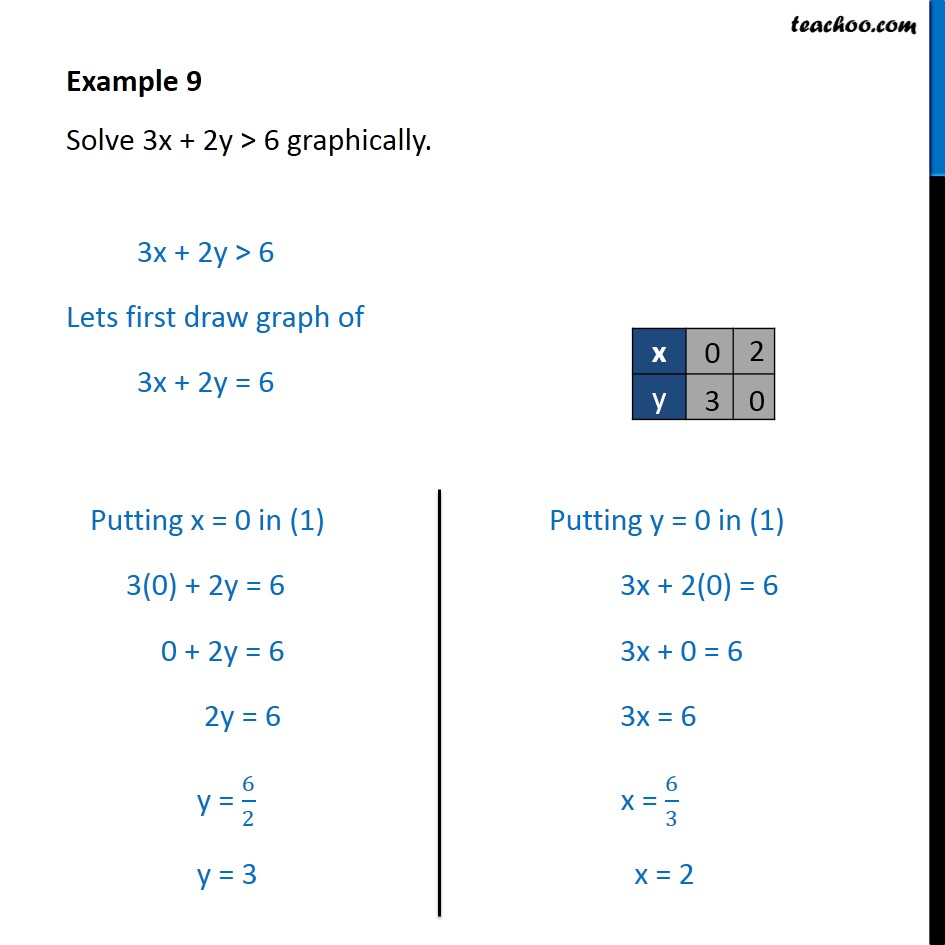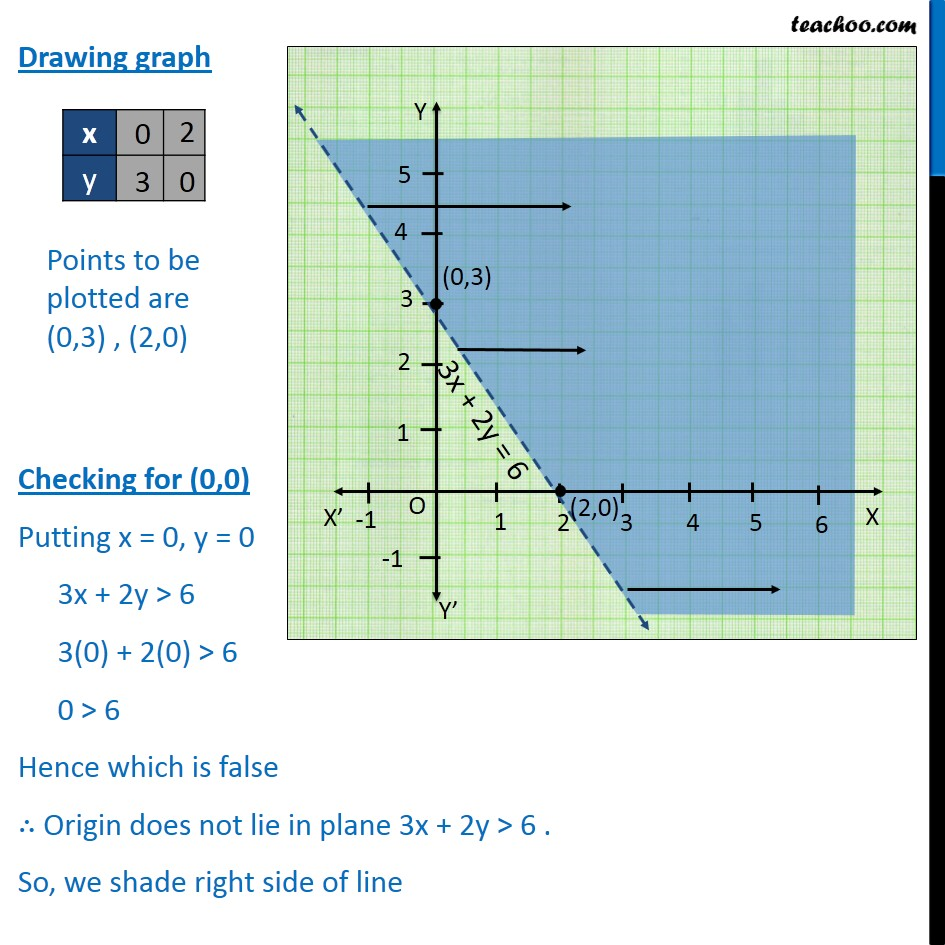Examples

Chapter 5 Class 11 Linear Inequalities
Serial order wiseLearn in your speed, with individual attention - Teachoo Maths 1-on-1 Class

### Transcript

Question 1 Solve 3x + 2y > 6 graphically. 3x + 2y > 6 Lets first draw graph of 3x + 2y = 6 Drawing graph Checking for (0,0) Putting x = 0, y = 0 3x + 2y > 6 3(0) + 2(0) > 6 0 > 6 Hence which is false ∴ Origin does not lie in plane 3x + 2y > 6 . So, we shade right side of line